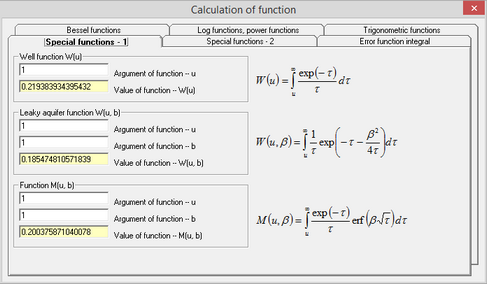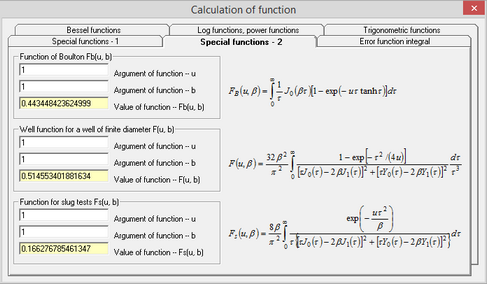﻿ Help > Functions

# Functions

The dialog window "Functions" assists in calculations of values for functions that are commonly used in hydrogeology.

The dialog window is opened by "Help > Functions...".Tab examples of the dialog window "Functions".

Explanatory table

 Tab "Special functions - 1" The most common special functions in drawdown analytical solutions: well function, leaky aquifer well function, M(u, β) Tab "Special functions - 2" Function of Bolton, Well function for a well of finite diameter, F(u, β) function for slug tests Tab "Error function (erfc)" Calculates error function (erf) and complimentary error function (erfc) Tab "Bessel functions" Calculates the main functions of Bessel Tab "Log functions, power functions" Calculates logarithmic, power and other axillary functions Tab "Trigonometric functions" Calculates trigonometric, hyperbolic, reverse trigonometric and reverse hyperbolic functions in rad and degrees.